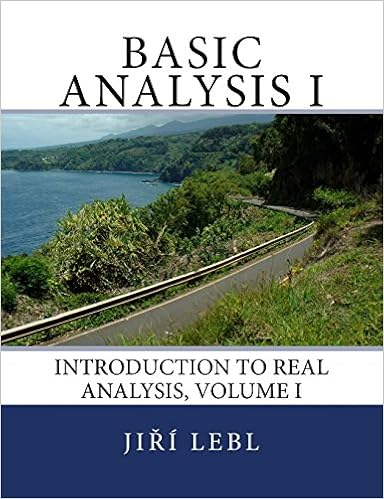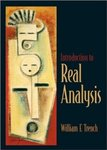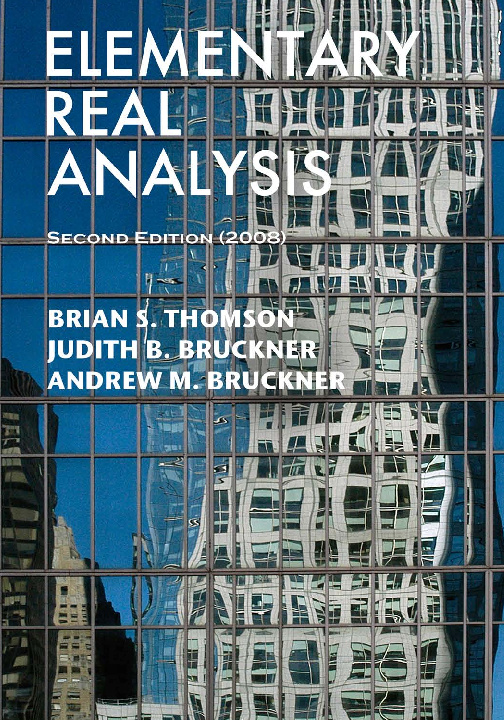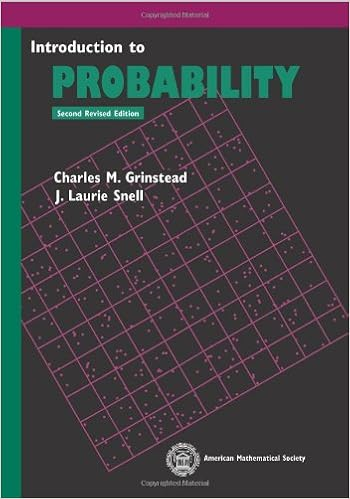# Mathematics

This guide is designed to assist faculty and students access scholarly Mathematics resources

#### ProofwritingTed Sundstrom, Mathematical Reasoning: Writing and Proof

This textbook is designed for the first course in the college mathematics curriculum that introduces students to the process of constructing and writing proofs. Previous versions of this textbook were published by Pearson Education, Inc. The current textbook is a revision of the second edition.

Approved by the American Institute of Mathematics.Richard Hammack, Book of Proof

Designed for the typical bridge course that follows calculus and introduces the students to the language and style of more theoretical mathematics, Book of Proof has 13 chapters grouped into four sections: (I) Fundamentals, (II) How to Prove Conditional Statements, (III) More on Proof, (IV) Relations, Functions, and Cardinality. [...]

Approved by the American Institute of Mathematics.
Review available from the MAA and the Open Textbook Library.Joe Fields, A Gentle Introduction to the Art of Mathematics

This text covers several topics in the foundations of mathematics (logic, sets, relations, functions and cardinality) and introduces the reader to many techniques of mathematical proof (direct, indirect, contradiction, contrapositive, mathematical induction, combinatorial proofs and magic). There are amusing quotations at the start of each chapter.

Approved by the American Institute of Mathematics.
Reviews available from the Open Textbook Library.

#### Abstract AlgebraTom Judson, Abstract Algebra: Theory and Applications

The first half of the book presents group theory, through the Sylow theorems, with enough material for a semester-long course. The second half is suitable for a second semester and presents rings, integral domains, Boolean algebras, vector spaces, and fields, concluding with Galois Theory.

Approved by the American Institute of Mathematics.
Review available from College Open Textbooks and the MAA.Edwin H. Connell, Elements of Abstract and Linear Algebra

The five chapters deal with fundamentals, groups, rings, matrix rings, and linear algebra. Despite the small size there is enough material for a full year. Many of the easy proofs are left as exercises. An instructor using this book should expect to add examples and build on the structure provided.

Approved by the American Institute of Mathematics.Frederick M. Goodman, Algebra: Abstract and Concrete

Algebra: Abstract and Concrete provides a thorough introduction to "modern'' or "abstract'' algebra at a level suitable for upper-level undergraduates and beginning graduate students. The book addresses the conventional topics: groups, rings, fields, and linear algebra, with symmetry as a unifying theme.

Approved by the American Institute of Mathematics.

#### Real AnalysisRobert Rogers and Eugene Boman, How We Got from There to Here: A Story of Real Analysis

This text for a semester course portrays real analysis in the context of its historical development. It is written in a direct style aimed at students and not instructors. A student using the book is guided to understand and prove much of the actual mathematical content through the more than 200 problems that are embedded within the narrative and not placed at the end of sections as in most textbooks. [...]

Approved by the American Institute of Mathematics.
Reviews available from the MAA and the Open Textbook Library.Jiří Lebl, Basic Analysis: Introduction to Real Analysis

This textbook is a one-semester course in basic analysis. A prerequisite for the course is a basic proof course. It should be possible to use the book for both a basic course for students who do not necessarily wish to go to graduate school, but also as a first semester of a more advanced course that also covers topics such as metric spaces. [...]

Approved by the American Institute of Mathematics.
Reviews available from the MAA and the Open Textbook Library.William F. Trench, Introduction to Real Analysis

Using a clear and informal approach, this book introduces readers to a rigorous understanding of mathematical analysis and presents challenging math concepts as clearly as possible. This book is intended for those who want to gain an understanding of mathematical analysis and challenging mathematical concepts.

Approved by the American Institute of Mathematics.Brian S. Thomson, Judith B. Bruckner, and Andrew M. Bruckner, Elementary Real Analysis

This book is the second edition of an undergraduate level Real Analysis textbook formerly published by Prentice Hall (Pearson) in 2001. It is designed to be a user-friendly text that is suitable for a one year course. [...]

Approved by the American Institute of Mathematics.
Review available from The UMAP Journal.

#### Probability